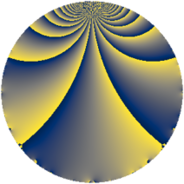# Properties

 Label 725.2.bLevel $725$ Weight $2$ Character orbit 725.b Rep. character $\chi_{725}(349,\cdot)$ Character field $\Q$ Dimension $42$ Newform subspaces $7$ Sturm bound $150$ Trace bound $9$

# Related objects

## Defining parameters

 Level: $$N$$ $$=$$ $$725 = 5^{2} \cdot 29$$ Weight: $$k$$ $$=$$ $$2$$ Character orbit: $$[\chi]$$ $$=$$ 725.b (of order $$2$$ and degree $$1$$) Character conductor: $$\operatorname{cond}(\chi)$$ $$=$$ $$5$$ Character field: $$\Q$$ Newform subspaces: $$7$$ Sturm bound: $$150$$ Trace bound: $$9$$ Distinguishing $$T_p$$: $$2$$, $$3$$

## Dimensions

The following table gives the dimensions of various subspaces of $$M_{2}(725, [\chi])$$.

Total New Old
Modular forms 82 42 40
Cusp forms 70 42 28
Eisenstein series 12 0 12

## Trace form

 $$42 q - 34 q^{4} - 8 q^{6} - 46 q^{9} + O(q^{10})$$ $$42 q - 34 q^{4} - 8 q^{6} - 46 q^{9} + 4 q^{11} + 4 q^{14} + 18 q^{16} + 12 q^{19} + 12 q^{21} + 56 q^{24} - 24 q^{26} - 6 q^{29} + 12 q^{31} + 56 q^{34} + 34 q^{36} - 40 q^{39} - 24 q^{41} + 36 q^{44} + 16 q^{46} - 26 q^{49} - 12 q^{51} + 44 q^{54} - 80 q^{56} + 36 q^{59} - 36 q^{61} + 26 q^{64} - 12 q^{66} - 40 q^{69} - 4 q^{71} + 36 q^{74} + 20 q^{76} + 20 q^{79} + 106 q^{81} - 60 q^{84} + 48 q^{86} - 28 q^{89} - 44 q^{91} - 64 q^{94} - 52 q^{96} + 60 q^{99} + O(q^{100})$$

## Decomposition of $$S_{2}^{\mathrm{new}}(725, [\chi])$$ into newform subspaces

Label Dim $A$ Field CM Traces $q$-expansion
$a_{2}$ $a_{3}$ $a_{5}$ $a_{7}$
725.2.b.a $2$ $5.789$ $$\Q(\sqrt{-1})$$ None $$0$$ $$0$$ $$0$$ $$0$$ $$q+iq^{2}+q^{4}+2iq^{7}+3iq^{8}+3q^{9}+\cdots$$
725.2.b.b $4$ $5.789$ $$\Q(\zeta_{8})$$ None $$0$$ $$0$$ $$0$$ $$0$$ $$q+(\zeta_{8}+\zeta_{8}^{2})q^{2}+(\zeta_{8}+\zeta_{8}^{2})q^{3}+(-1+\cdots)q^{4}+\cdots$$
725.2.b.c $4$ $5.789$ $$\Q(\zeta_{8})$$ None $$0$$ $$0$$ $$0$$ $$0$$ $$q+\zeta_{8}q^{2}+(-\zeta_{8}-\zeta_{8}^{2})q^{3}+(-1-\zeta_{8}^{3})q^{4}+\cdots$$
725.2.b.d $6$ $5.789$ 6.0.350464.1 None $$0$$ $$0$$ $$0$$ $$0$$ $$q+\beta _{5}q^{2}+(\beta _{2}-\beta _{4})q^{3}+(-2-\beta _{3}+\cdots)q^{4}+\cdots$$
725.2.b.e $6$ $5.789$ 6.0.350464.1 None $$0$$ $$0$$ $$0$$ $$0$$ $$q-\beta _{4}q^{2}+(\beta _{2}-\beta _{4})q^{3}+\beta _{1}q^{4}+(-1+\cdots)q^{6}+\cdots$$
725.2.b.f $10$ $5.789$ $$\mathbb{Q}[x]/(x^{10} + \cdots)$$ None $$0$$ $$0$$ $$0$$ $$0$$ $$q+\beta _{1}q^{2}+(-\beta _{4}+\beta _{7})q^{3}+(-1+\beta _{2}+\cdots)q^{4}+\cdots$$
725.2.b.g $10$ $5.789$ $$\mathbb{Q}[x]/(x^{10} + \cdots)$$ None $$0$$ $$0$$ $$0$$ $$0$$ $$q+\beta _{1}q^{2}+(\beta _{2}+\beta _{7})q^{3}+(-1+\beta _{5}+\cdots)q^{4}+\cdots$$

## Decomposition of $$S_{2}^{\mathrm{old}}(725, [\chi])$$ into lower level spaces

$$S_{2}^{\mathrm{old}}(725, [\chi]) \cong$$ $$S_{2}^{\mathrm{new}}(145, [\chi])$$$$^{\oplus 2}$$